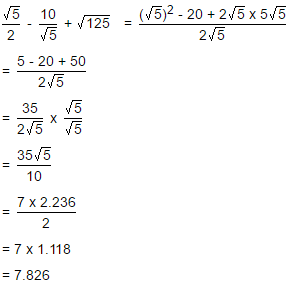Courses

# Test: Square Root And Cube Root- 2

## 10 Questions MCQ Test IBPS PO Mains - Study Material, Online Tests, Previous Year | Test: Square Root And Cube Root- 2

Description
This mock test of Test: Square Root And Cube Root- 2 for Quant helps you for every Quant entrance exam. This contains 10 Multiple Choice Questions for Quant Test: Square Root And Cube Root- 2 (mcq) to study with solutions a complete question bank. The solved questions answers in this Test: Square Root And Cube Root- 2 quiz give you a good mix of easy questions and tough questions. Quant students definitely take this Test: Square Root And Cube Root- 2 exercise for a better result in the exam. You can find other Test: Square Root And Cube Root- 2 extra questions, long questions & short questions for Quant on EduRev as well by searching above.
QUESTION: 1

### Practice Quiz or MCQ (Multiple Choice Questions) with solutions are available for Practice, which would help you prepare for "Square Root and Cube Root" under Quantitative Aptitude. You can practice these practice quizzes as per your speed and improvise the topic. The same topic is covered under various competitive examinations like - CAT, GMAT, Bank PO, SSC and other competitive examinations.   Q. The cube root of .000216 is:

Solution: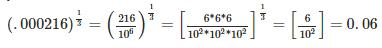QUESTION: 2

### What should come in place of both x in the equation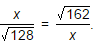Solution: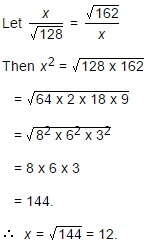QUESTION: 3

### The least perfect square, which is divisible by each of 21, 36 and 66 is:

Solution:

L.C.M. of 21, 36, 66 = 2772.

Now, 2772 = 2 x 2 x 3 x 3 x 7 x 11

To make it a perfect square, it must be multiplied by 7 x 11.

So, required number = 22 x 32 x 72 x 112 = 213444

QUESTION: 4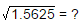Solution: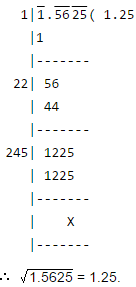QUESTION: 5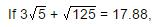then what will be the value of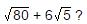Solution: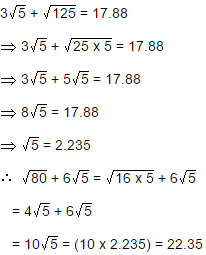QUESTION: 6

If a = 0.1039, then the value of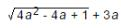is:

Solution: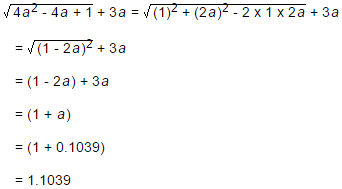QUESTION: 7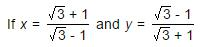then the value of (x2 + y2) is:

Solution: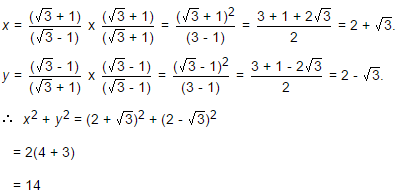QUESTION: 8

A group of students decided to collect as many paise from each member of group as is the number of members. If the total collection amounts to Rs. 59.29, the number of the member is the group is:

Solution: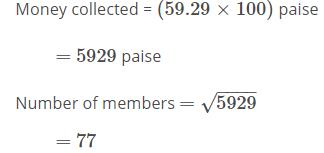QUESTION: 9

The square root of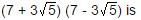Solution: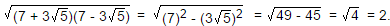QUESTION: 10

If √5 = 2.236, then the value of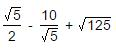is equal to:

Solution: